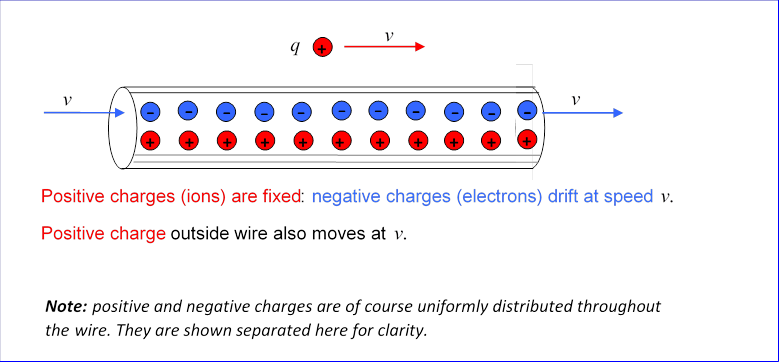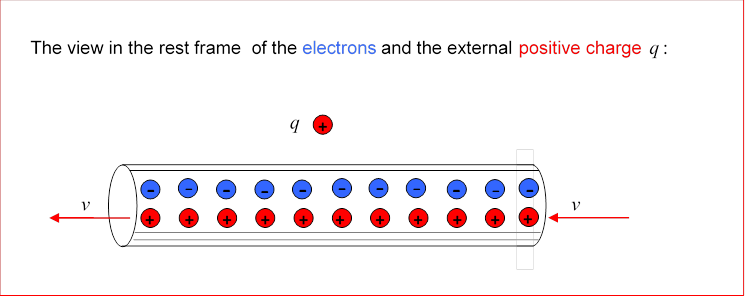# How Relativity Connects Electric and Magnetic Fields

Michael Fowler, University of Virginia

## A Magnetic Puzzle…

Suppose we have an infinitely long straight wire, having a charge density of electrons of $−λ$ coulombs per meter, all moving at speed $v$ to the right (recall typical speeds are centimeters per minute) and a neutralizing fixed background of positive charge, also of course $λ$ coulombs per meter.  The current in the wire has magnitude $I=λv$ (and actually is flowing to the left, since the moving electrons carry negative charge).

Suppose also that a positive charge $q$ is outside the wire, a distance $r$ from the axis, and this outside charge is moving at the same exact velocity as the electrons in the wire.What force does the positive charge $q$ feel?

The wire is electrically neutral, since it contains equal densities of positive and negative charges, both uniformly distributed throughout the wire (the illustration above is of course schematic).  So $q$ feels no electrical force.

However, since $q$ is moving, it will feel a magnetic force, $F → mag =q v → × B → .$

The magnetic field lines are vertical circles centered along the axis of the wire, the field being into the page where the charge is (remember the current is to the left) and of magnitude

$B= μ 0 I 2πr = μ 0 λv 2πr$

so the force on the charge is of magnitude

$F= q μ 0 λ v 2 2πr$

and is directed away from the wire, so the charge will accelerate away from the wire.

Now let us examine the same physical system in the frame of reference in which the charge is initially at rest.  In that frame, the electrons are also at rest, but the positive background charge is flowing at $v:$What force does $q$ feel in this frame?

Since $q$ is at rest, it cannot feel a magnetic force: such forces depend linearly on speed!

Yet it looks as if it can’t feel an electric force either, because the positive and negative charges in the wire have equal densities, right?  This leads to the conclusion that $q$ feels no force at all in this frame, so it won’t accelerate away from the wire, as it did in the other frame.   This is of course nonsense$—$so where did we go wrong?

## … Solved Electrically!

The mistake was in ignoring the relativistic Fitzgerald-Lorentz contraction, even though the velocities involved are millimeters per second!   In the frame in which the wire is at rest, the positive and negative charge densities exactly balance, otherwise there will be extra electrostatic fields that the electrons will quickly move to neutralize.  However, this necessarily means that the densities cannot balance exactly in the frame in which the drifting electrons are at rest.

In the electrons’ rest frame, the positive charges, which had density λ coulombs per meter in their rest frame, are moving at speed v, so relativistic contraction will increase their density to:

$λ 1− v 2 / c 2 ≅λ+ λ v 2 2 c 2 .$

On the other hand, in this electron frame the electrons are at rest, so their density is actually less than it was in the frame of the wire, it has decreased by $λ v 2 /2 c 2 .$

The net effect is that the wire, electrically neutral in the lab frame, has a positive charge density $λ v 2 / c 2$ in the frame of the moving electrons (and the outside charge).  Recall Gauss’s Law relating the total electric field flux through a surface to the enclosed charge $Q encl :$

$∫ E → .d A → = Q encl / ε 0 .$

Exercise: use this to verify that the electric field from an infinite line of charge $λ$ coulombs per meter has magnitude $λ/2πr ε 0$ at distance $r$ from the line charge.

From the exercise result, the electrostatic force on the charge $q$ is:

$F=qE=qλ v 2 c 2 1 2πr ε 0 = qλ v 2 2πr 1 ε 0 c 2 = qλ v 2 2πr μ 0$

where in the last step we used $1/ c 2 = μ 0 ε 0 .$

This purely electrical force is identical in magnitude to the purely magnetic force in the other frame!  So observers in the two frames will agree on the rate at which the particle accelerates away from the wire, but one will call the accelerating force magnetic, the other electric.  We are forced to the conclusion that whether a particular force on an actual particle is magnetic or electric, or some mixture of both, depends on the frame of reference$—$so the distinction is rather artificial.

A much more complete analysis of the fields from moving charges can be found in Purcell, Electricity and Magnetism, McGraw Hill, 1985.  We have chosen the simplest possible example to illustrate the basic point that electric and magnetic fields are frame-dependent concepts.